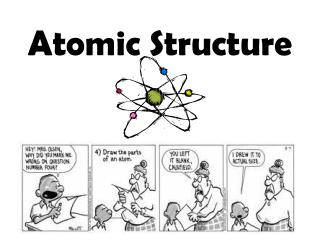DownloadDownload PresentationAtomic Structure

# Atomic Structure

Download Presentation## Atomic Structure

- - - - - - - - - - - - - - - - - - - - - - - - - - - E N D - - - - - - - - - - - - - - - - - - - - - - - - - - -
##### Presentation Transcript

1. Atomic Structure

2. Atom • Basic unit of matter • Made of subatomic particles

3. Element • Substance made of same type of atom

4. Protons • Positive Charge • Located in the nucleus • Mass = 1 amu • Helps make up the mass of an atom • # of protons never changes for an element • Same as atomic number

5. Neutrons • No Charge • Located in the nucleus • Mass = 1 amu • Helps make up the mass of an atom • Can differ in atoms of the same element How to Calculate Neutrons Mass # - Atomic # = Neutrons

6. Electrons • Negative Charge • Located in the electron cloud • Surrounds the nucleus • Move very fast! • Mass = 0 amu • Does not really count towards an atom’s mass • In neutral atoms, electrons = protons

7. Atomic Number • Special for every element • Tells # of protons (and electrons) • Located on the periodic table (whole number)

8. Atomic Mass • Located on periodic table • Has decimals • Average mass of the element’s isotopes

9. Mass Number • Protons + Neutrons • Whole Number (rounded atomic mass)

10. Practice • How many protons does nitrogen have?

11. Practice • What is the atomic mass of calcium?

12. Practice • What is the mass number of calcium?

13. Practice • How many neutrons are in chlorine?

14. Practice • Name of Element: • Atomic Number: • Atomic Mass: • Mass Number: • Protons: • Neutrons: • Electrons:

15. Practice • Name of Element: • Atomic Number: • Atomic Mass: • Mass Number: • Protons: • Neutrons: • Electrons:

16. Practice • Name of Element: • Atomic Number: • Atomic Mass: • Mass Number: • Protons: • Neutrons: • Electrons:

17. Just How Small Are Atoms?

18. Isotopes • Same element with different numbers of neutrons; therefore, different masses • EX: Carbon-12 Carbon-14 612 C6 14 C

19. Practice • How many neutrons in Potassium-39 Potassium-41 Fluorine-19 Fluorine-18

20. Practice • Ni-60 • Protons? • Electrons? • Mass number? • Neutrons?

21. Practice • Magnesium-26 • Protons? • Electrons? • Mass number? • Neutrons?

22. Practice • 1529 P • Protons? • Electrons? • Mass number? • Neutrons?

23. Ions • Charged atom • Gain or lose 1 or more electrons • Proton number does not change

24. Practice How many electrons in a neutral atom of Na? How many protons in Na? How many electrons in Na +1

25. Practice How many electrons in a neutral atom of S? How many protons in S? How many electrons in S -2?